Two resistors of resistance R1 and R2 having R1 > R2 are connected in parallel. For equivalent resistance R, the correct statement is :

(1) $R>{R}_{1}+{R}_{2}$

(2) ${R}_{1}

(3) ${R}_{2}

(4) R < R1

Concept Questions :-

Combination of resistors
High Yielding Test Series + Question Bank - NEET 2020

Difficulty Level:

A wire of resistance R is divided into 10 equal parts. These parts are connected in parallel, the equivalent resistance of such connection will be :

(1) 0.01 R

(2) 0.1 R

(3) 10 R

(4) 100 R

Concept Questions :-

Combination of resistors
High Yielding Test Series + Question Bank - NEET 2020

Difficulty Level:

The current in the adjoining circuit will be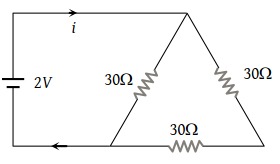(1) $\frac{1}{45}ampere$

(2) $\frac{1}{15}ampere$

(3) $\frac{1}{10}ampere$

(4) $\frac{1}{5}ampere$

Concept Questions :-

Combination of resistors
High Yielding Test Series + Question Bank - NEET 2020

Difficulty Level:

The reading of the ammeter as per figure shown is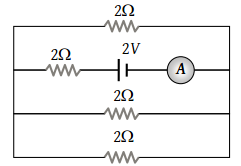(1) $\frac{1}{8}A$

(2) $\frac{3}{4}A$

(3) $\frac{1}{2}A$

(4) 2 A

Concept Questions :-

Combination of resistors
High Yielding Test Series + Question Bank - NEET 2020

Difficulty Level:

Three resistors each of 2 ohm are connected together in a triangular shape. The resistance between any two vertices will be

(1) 4/3 ohm

(2) 3/4 ohm

(3) 3 ohm

(4) 6 ohm

Concept Questions :-

Combination of resistors
High Yielding Test Series + Question Bank - NEET 2020

Difficulty Level:

There are n similar conductors each of resistance R. The resultant resistance comes out to be x when connected in parallel. If they are connected in series, the resistance comes out to be :

(1) x/n2

(2) n2x

(3) x/n

(4) nx

Concept Questions :-

Combination of resistors
High Yielding Test Series + Question Bank - NEET 2020

Difficulty Level:

Equivalent resistance between A and B will be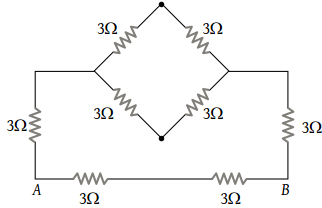(1) 2 ohm

(2) 18 ohm

(3) 6 ohm

(4) 3.6 ohm

Concept Questions :-

Combination of resistors
High Yielding Test Series + Question Bank - NEET 2020

Difficulty Level:

A current of 2 A flows in a system of conductors as shown. The potential difference $\left({V}_{A}-{V}_{B}\right)$ will be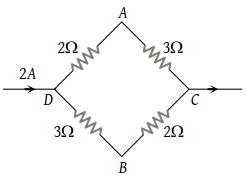(1) +2 V

(2) +1 V

(3) –1 V

(4) –2 V

Concept Questions :-

Kirchoff's voltage law
High Yielding Test Series + Question Bank - NEET 2020

Difficulty Level:

Referring to the figure below, the effective resistance of the network is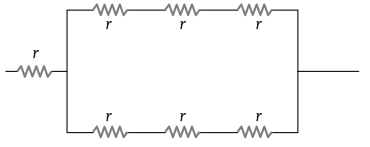(1) 2r

(2) 4r

(3) 10r

(4) 5r/2

Concept Questions :-

Combination of resistors
High Yielding Test Series + Question Bank - NEET 2020

Difficulty Level:

Two resistances are joined in parallel whose resultant is $\frac{6}{8}$ ohm. One of the resistance wire is broken and the effective resistance becomes 2Ω. Then the resistance in ohm of the wire that got broken was

(1) 3/5

(2) 2

(3) 6/5

(4) 3

Concept Questions :-

Combination of resistors